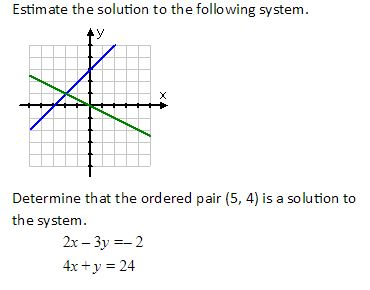#### MAT-HS.A-REI.06

 MAT-HS Targeted Standards(A) Concept: Algebra(REI) Domain: Reasoning with Equations and InequalitiesCluster: Solve systems of equations MAT-HS.A-REI.06 Solve systems of linear equations exactly and approximately, focusing on pairs of linear equations in two variables.

## Student Learning Targets:

• I can
• I can

• I can
• I can

### Skills (Performance) Targets

• I can solve systems of equations using graphing (exactly and approximating).
• I can solve systems of equations using substitution.
• I can solve systems of equations using linear combination (or elimination).
• I can explain when there is one solution, no solution, or infinitely many solutions that may arise from a system.

• I can
• I can

## Proficiency Scale

 Score Description Sample Activity 4.0 (advanced) In addition to achieving level 3.0 content, the student makes in-depth inferences and applications that go beyond what was taught Solve a  3x3 system of equations 2x-y+5z=16x-3y+2z=5x+2y+z=7 3.5 In addition to Score 3.0 performance, the student demonstrates in-depth inferences and applications regarding the more complex content with partial success. 3.0 (proficient) The student can: solve systems of equations using graphing (exactly and approximating) solve systems of equations using substitution solve systems of equations using linear combination (or elimination) explain when there is one solution, no solution, or infinitely many solutions that may arise from a system. The student exhibits no major errors or omissions. Solve the following system of equations using your preferred method. x – 2y = –9 x + 3y = 16 Explain when one solution, no solutions, and infinitely many solutions will arise. 2.5 The student demonstrates no major errors or omissions regarding the simpler details and processes (Score 2.0 content) and partial knowledge of the more complex ideas and processes (Score 3.0 content). 2.0 (progressing) There are no major errors or omissions regarding the simpler details and processes as the student can: recognize and recall basic terminology. such as: linear combination (elimination) substitution solution infinitely many solutions no solution system of equations ordered pair determine if an ordered pair is a solution to a system. approximate a solution to a system of linear equations that are graphed. However, the student exhibits major errors or omissions regarding the more complex ideas and processes.1.5 The student demonstrates partial knowledge of the simpler details and processes (Score 2.0 content) but exhibits major errors or omissions regarding the more complex ideas and procedures (Score 3.0 content). 1.0 (beginning) With help, a partial understanding of some of the simpler details and processes and some of the more complex ideas and processes. - 0.5 With help, the student demonstrates a partial understanding of some of the simpler details and processes (Score 2.0 content) but not the more complex ideas and processes (Score 3.0 content).

## Resources

 Web Vocab linear combination elimination method substitution method